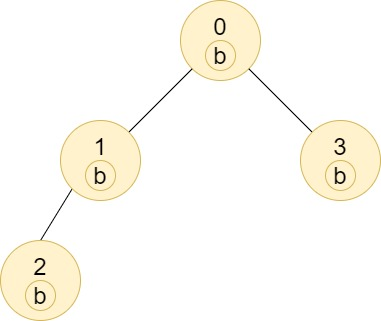# 1519. Number of Nodes in the Sub-Tree With the Same Label ⭐

Medium
You are given a tree (i.e. a connected, undirected graph that has no cycles) consisting of `n` nodes numbered from `0` to `n - 1` and exactly `n - 1` `edges`. The root of the tree is the node `0`, and each node of the tree has a label which is a lower-case character given in the string `labels` (i.e. The node with the number `i` has the label `labels[i]`).
The `edges` array is given on the form `edges[i] = [ai, bi]`, which means there is an edge between nodes `ai` and `bi` in the tree.
Return an array of size `n` where `ans[i]` is the number of nodes in the subtree of the `ith` node which have the same label as node `i`.
A subtree of a tree `T` is the tree consisting of a node in `T` and all of its descendant nodes.
Example 1:Input: n = 7, edges = [[0,1],[0,2],[1,4],[1,5],[2,3],[2,6]], labels = "abaedcd"
Output: [2,1,1,1,1,1,1]
Explanation: Node 0 has label 'a' and its sub-tree has node 2 with label 'a' as well, thus the answer is 2. Notice that any node is part of its sub-tree.
Node 1 has a label 'b'. The sub-tree of node 1 contains nodes 1,4 and 5, as nodes 4 and 5 have different labels than node 1, the answer is just 1 (the node itself).
Example 2:Input: n = 4, edges = [[0,1],[1,2],[0,3]], labels = "bbbb"
Output: [4,2,1,1]
Explanation: The sub-tree of node 2 contains only node 2, so the answer is 1.
The sub-tree of node 3 contains only node 3, so the answer is 1.
The sub-tree of node 1 contains nodes 1 and 2, both have label 'b', thus the answer is 2.
The sub-tree of node 0 contains nodes 0, 1, 2 and 3, all with label 'b', thus the answer is 4.
Example 3:Input: n = 5, edges = [[0,1],[0,2],[1,3],[0,4]], labels = "aabab"
Output: [3,2,1,1,1]
Constraints:
• `1 <= n <= 10^5`
• `edges.length == n - 1`
• `edges[i].length == 2`
• `0 <= ai, bi < n`
• `ai != bi`
• `labels.length == n`
• `labels` is consisting of only of lowercase English letters.

### 解題

⭐不要做一棵樹，想成graph⭐
Runtime: 278 ms, faster than 100%
Memory Usage: 48.7 MB, less than 25%
func countSubTrees(n int, edges [][]int, labels string) []int {
ans := make([]int, n)
graph := make([][]int, n) // 紀錄各個節點的鄰邊
for _, edge := range edges {
u := edge
v := edge
graph[u] = append(graph[u], v)
graph[v] = append(graph[v], u)
}
var dfs func(int, int) []int
dfs = func(u int, parent int) []int {
count := make([]int, 26)
for _, v := range graph[u] { // 加總子節點的label
if v == parent { continue } // 不會往上(parent)數
childCount := dfs(v, u)
for i:=0; i<26; i++ {
count[i] += childCount[i]
}
}
count[labels[u] - 'a']++ // 把自己的label也加上去
ans[u] = count[labels[u] - 'a'] // 紀錄這個節點的答案
return count
}
dfs(0, -1)
return ans
}